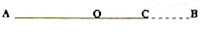## Friday, June 15, 2007

### MCQ on Moment of Inertia of a Rod

Moment of inertia is a scalar quantity. So you can add and subtract moment of inertia as you do in the case of scalar quantities, but you should remember that the moment of inertia depends on the axis chosen. You cannot add (or subtract) the moments of inertia of bodies about different axes, but you can add (or subtract) moments of inertia if the axis is the same. Now consider the following MCQ which will make things clear:

A thin uniform rod AB of mass 12M and length L has moment of inertia I about an axis passing through its mid point O perpendicular to its length. If a portion CB of length (L/4) is cut off from the end of the rod, the moment of inertia of the remaining portion AC about the original axis through O will be(a) (9/16)I

(b) (¾)I

(c) (13/15)I

(d) (41/48)I

(e) (51/64)I

You have to subtract the moment of inertia of the portion CB from the moment of inertia of the original rod AB to obtain the moment of inertia of the remaining portion AC ( since all moments of inertia are about the same axis passing normally through O).

Moment of inertia (I) of original rod AB = 12ML2/12 = ML2 (The mass of the rod in this problem is given as 12M to make the terms simple).

Moment of inertia of the portion CB of mass 3M and length (L/4) is (by applying the parallel axis theorem) 3M(L/4)2/12 + 3M(3L/8)2 = (7/16)ML2

[The first term is the moment of inertia of portion CB about an axis through its centre and the second term is the product of its mass and the square of the distance between the two parallel axes].

Therefore, moment of inertia of the remaining portion AC = ML2(7/16)ML2 = (9/16)ML2 = (9/16)I.

[Alternatively, you can treat the portion AC of the rod to be made of the portions AO and OC and add their moments of inertia about O to arrive at the answer, remembering that the moment of inertia of a thin rod of mass ‘m’ and length ‘l’ about a normal axis through one end is ml2/3. The moment of inertia of the portion AC of the rod is therefore 6M(L/2)2/3 + 3M(L/4)2/3 = ML2/2 + ML2/16 = (9/16)ML2 = (9/16)I].